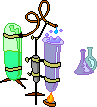Dr. Herrick's CH222 Pressure Quiz 1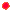Periodic trends and bond models Copyright D. Herrick20 Questions from past exams. Practice for speed.  Aim for 2 minutes per problem.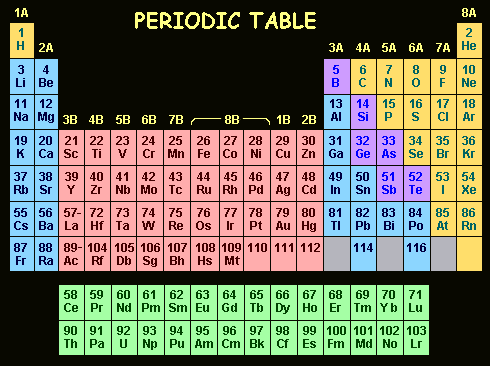1 Which of these atoms has the largest radius?
 A)  S B)  F C)  Cl D)  O E)  Ne
 2 Which of these atoms has the largest ionization energy?
 A)  S B)  F C)  Cl D)  O E)  Ne
 3 Which of these atoms has the smallest radius?
 A)  Na B)  Mg C)  K D)  Ca E)  Ba4 The electron configuration of the ion O2- is
 A) 1s2 2p6 B) 1s2 2s2 2p4 C) 1s2 2s2 2p6 3s2 D) 1s2 2s2 2p6 E) 1s2 2p6 3s2
 5 Which ion has the largest radius?
 A)  Cl- B)  S2- C)  P3- D)  Al3+ E)  Mg2+
 6 Atoms in Group 7A have the valence configuration
 A) -ns2 np7 B) -ns2 np5 C) -ns2 np3 D) -ns2 np2 E) -ns2 np6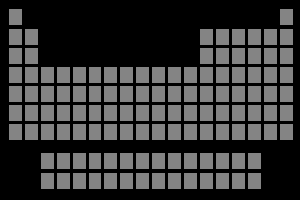7 Which of these atoms has the largest radius?
 A)  Mg B)  Cs C)  Na D)  K E)  Ba
 8 The total number of electrons in the ion Al3+ is
 A)  10 B)  12 C)  13 D)  15 E)  16
 9 Which atom has the largest ionization energy?
 A)  K B)  Cs C)  I D)  Ba E)  Cl10 Which bond is expected to have the highest ionic character?
 A)  Cl-F B)  I-F C)  Br-F D)  F-F
 11 Which is expected to have the highest degree of metallic bonding?
 A)  Si(s) B)  Ba(s) C)  S(s) D)  Ca(s)
 12 Which pair is least likely to form an ionic bond?
 A)  Na and S B)  Br and Mg C)  N and C D)  O and Cs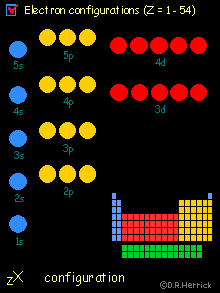13 The best description of the bonding between nitrogen and phosphorous atoms is
 A) ionic B) covalent C) polar covalent with the negative partial charge on N D) polar covalent with the negative partial charge on P E) metallic
 14 Which bond has the highest degree of covalent character?
 A)  Cl-P B)  Cl-S C)  Cl-Si D)  Cl-Mg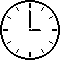15 Which solid has the least metallic bonding character?
 A)  K B)  Mg C)  Na D)  Al
 16 Which ionic solid has the largest lattice energy?
 A)   LiF B)   NaBr C)   CsCl D)  KI
 17 The correct order of increasing (smaller < larger) ionic radii is:
 A) Cl-  <  S2-  <  K+  <  Ca2+ B) K+ <  Ca2+ < Cl-  <  S2- C) S2-  <  Cl-  <  K+  <  Ca2+ D) Ca2+ <  K+ <  Cl-  <  S2- E) Ca2+ <  K+ <  S2-   <  Cl-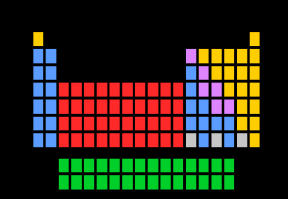18 Use the bond energies for H2 (436 kJ), Cl2 (242 kJ) and HCl (431 kJ) to estimate the enthalpy change in kJ for the reaction       H + Cl2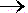HCl + Cl
 A) -152 B)  -189 C) 206 D) +189 E) 152
 19 Which ionic solid has the largest lattice energy?
 A)  KCl B)  KI C)  CsCl D)  CsI
20. Use the data (kJ) for ionization energies, electron affinities and heats of formation to find the lattice energy of Fe3O2(s) according to the equation

Fe2O3(s)2Fe3+(g)  + 3O2-(g)

 IE1(Fe) = 762 EA1(O) = -141 DHf (Fe2O3(s)) = -826 IE2(Fe) = 1562 EA2(O) =  746 DHf (Fe(g)) =  416 IE3(Fe) = 2957 DHf (O(g)) =  249
 A) 12571 B) 15604 C) 9332 D) 14782 E) 13854